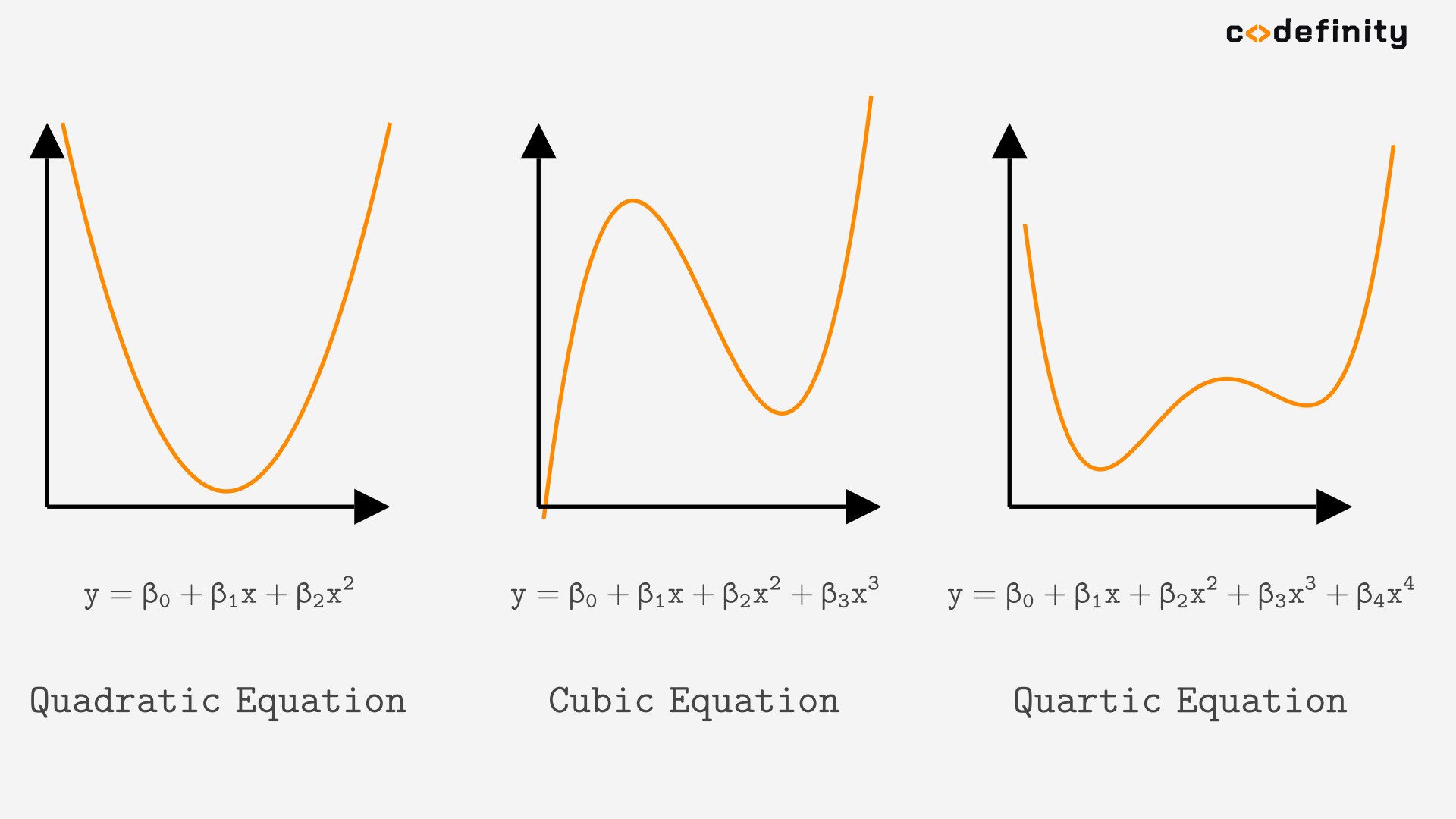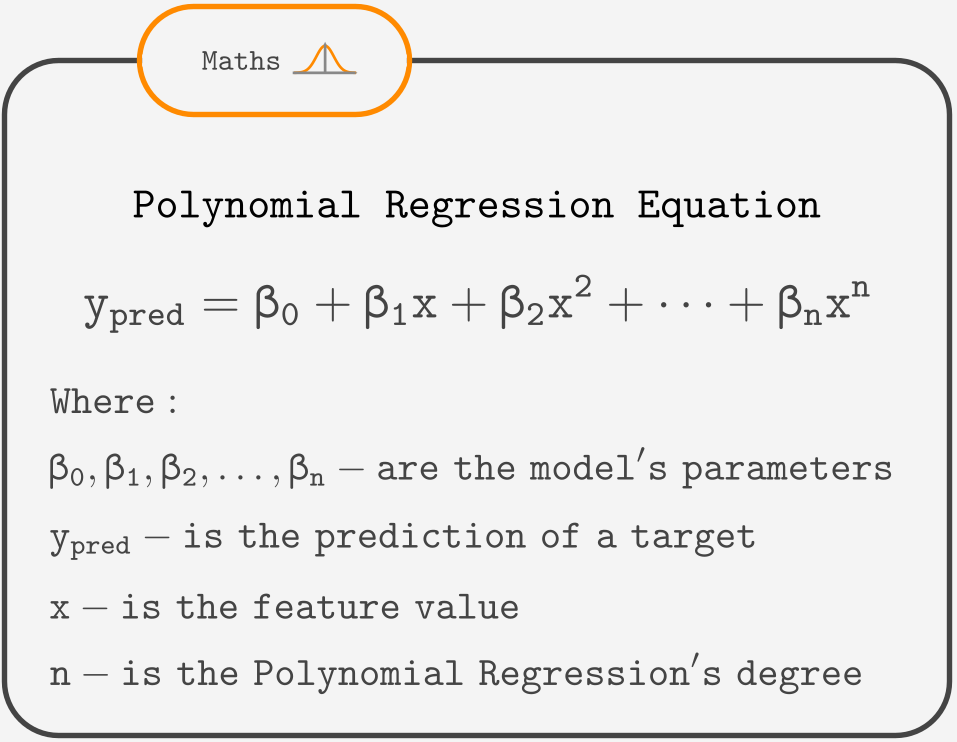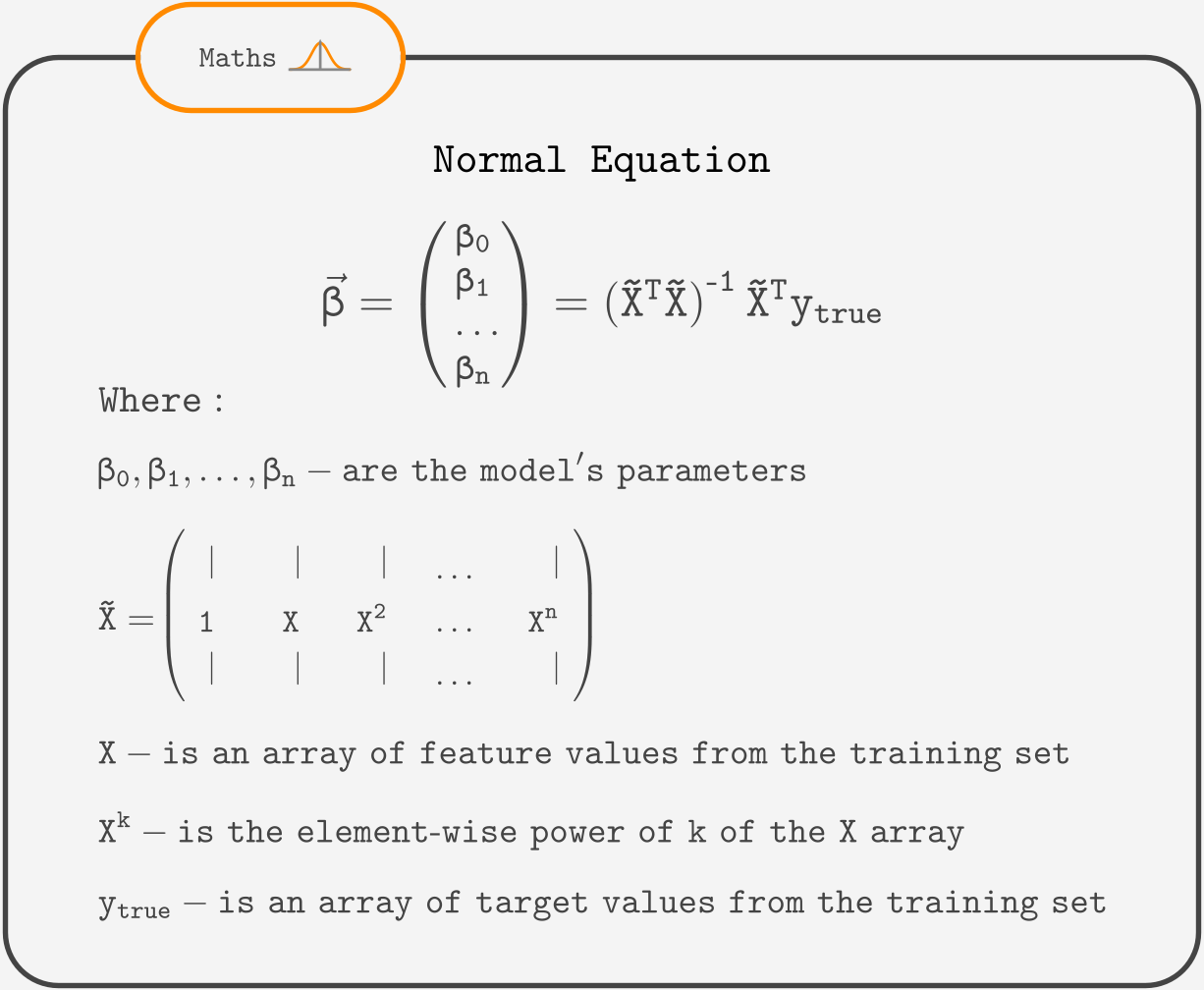Course Content

# Linear Regression with Python

Linear Regression with Python

##Polynomial Regression

In the previous chapter, we explored quadratic regression, which has the graph of a parabola. In the same way, we could add the to the equation to get the Cubic Regression that has a more complex graph. We could also add x⁴ and so on.## A degree of a Polynomial Regression

In general, it is called the polynomial equation and is the equation of Polynomial Regression. The highest power of x defines a degree of a Polynomial Regression in the equation. Here is an example

## n-degree Polynomial Regression

Considering n to be a whole number greater than two, we can write down the equation of an n-degree Polynomial Regression.## Normal Equation

And as always, parameters are found using the Normal Equation:## Polynomial Regression with multiple features

To create even more complex shapes, you can use the Polynomial Regression with more than one feature. But even with two features, 2-degree Polynomial Regression has quite a long equation.Most of the time, you won't need that complex model. Simpler models (like Multiple Linear Regression) usually describe the data well enough, and they are much easier to interpret, visualize and less computationally expensive.